# Flip-flop types ,their Conversion and Applications

Flip-flop is a circuit that maintains a state until directed by input to change the state. A basic flip-flop can be constructed using four-NAND or four-NOR gates.
Types of flip-flops:

1. RS Flip Flop
2. JK Flip Flop
3. D Flip Flop
4. T Flip Flop

Logic diagrams and truth tables of the different types of flip-flops are as follows:

## S-R Flip Flop :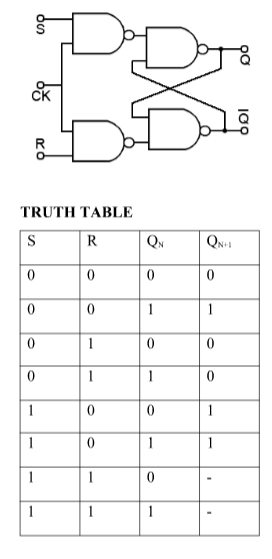## J-K Flip Flop: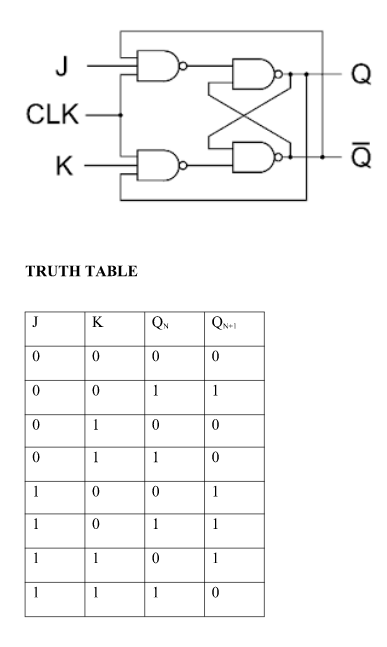## Conversion for FlipFlops :-

EXCITATION TABLE: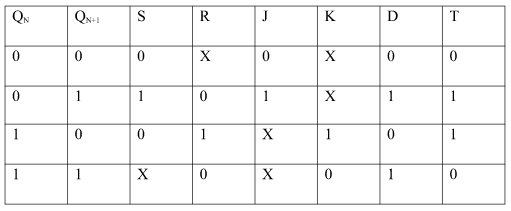Steps To Convert from One FlipFlop to Other :

Let there be required flipflop to be constructed using sub-flipflop:

1. Draw the truth table of required flipflop.
2. Write the corresponding outputs of sub-flipflop to be used from the excitation table.
3. Draw K-Maps using required flipflop inputs and obtain excitation functions for sub-flipflop inputs.
4. Construct logic diagram according to the functions obtained.

i) Convert SR To JK FlipFlop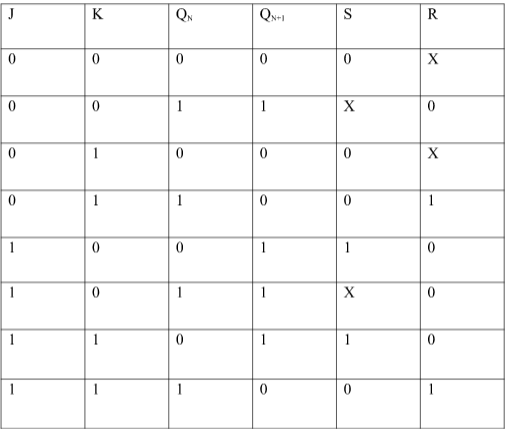ii) Convert SR To D FlipFlop: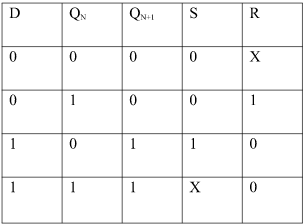Excitation Functions:
S = D
R = D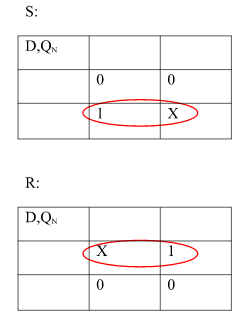Applications of Flip-Flops
These are the various types of flip-flops being used in digital electronic circuits and the applications of Flip-flops are as specified below.

• Counters
• Frequency Dividers
• Shift Registers
• Storage Registers
• Bounce elimination switch
• Data storage
• Data transfer
• Latch
• Registers
• Memory